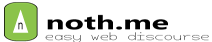# Power Query 笔记

1. Power Query动态汇总文件夹下多个Excel文件
点击“数据 ”– “从文件 ”– 从“文件夹 ”–保留”Content ”列– 选择“Content ”列，并点击“开始 ” – ”删除其他列 “。
点击“添加列 “– ”添加自定义列 “， 输入Excel.Workbook([Content],true) ，通过Excel.Workbook把Content 里的内容提取出来（指定True,实现了默认情况下将第一行作为标题，省去了后面提升和筛选标题行的操作。）。
点新建的列右的双箭头图标按钮，把所有字段扩展出来（有的可能要点几次筛选才能得到需要的数据）
经过筛选，即可看到，要合并的数据。
Step6 ：点击【确定】后即可得到最终的汇总结果。
将来数据更新后我们只需要在合并后的Excel表中点击【数据】→【连接】→【全部刷新】即可获得最新数据合并结果，一劳永逸。

2.‘Excel.CurrentWorkbook’ 列出工作簿中：定义了名称的表或公式定义了名称的表

3.孙新华教程

``````追加查询-汇总多个工作表【同一工作簿下】----

= List.Distinct(List.Combine(List.Transform(删除的其他列[Data],each Table.ColumnNames(_))))

=left(SUBSTITUTE(CELL("filename"),"[",""),Find("]",SUBSTITUTE(CELL("filename"),"[",""))-1)

Excel.CurrentWorkbook(){[Name="sxh"]}[Content]{0}[Column1]

= List.Distinct(List.Combine(List.Transform(删除的其他列1[Data],each Table.ColumnNames(_))))

Excel.CurrentWorkbook(){[Name="sxh"]}[Content]{0}[Column1]08

（1）添加自定义列Excel.Workbook([Content],true)
（2）自定义字段名= List.Distinct(List.Combine(List.Transform(删除的其他列1[Data],each Table.ColumnNames(_))))
(3)自动识别路径：

(4)替换路径Excel.CurrentWorkbook(){[Name="sxh"]}[Content]{0}[Column1]09

``````